# Pie Chart

A pie chart is a type of graph that represents data as a circle divided into slices. Each slice represents a proportion of the whole, and the size of the slice corresponds to the magnitude of the proportion. Use Pie charts for better display of percentages and fractions of a total.Transcript# Creating a slice Chart widget

• Under any App, go to reports
• Click + Report
• Select the list you want to get the data from.
• From the Chart Type, select Pie Chart
• Then continue to configure it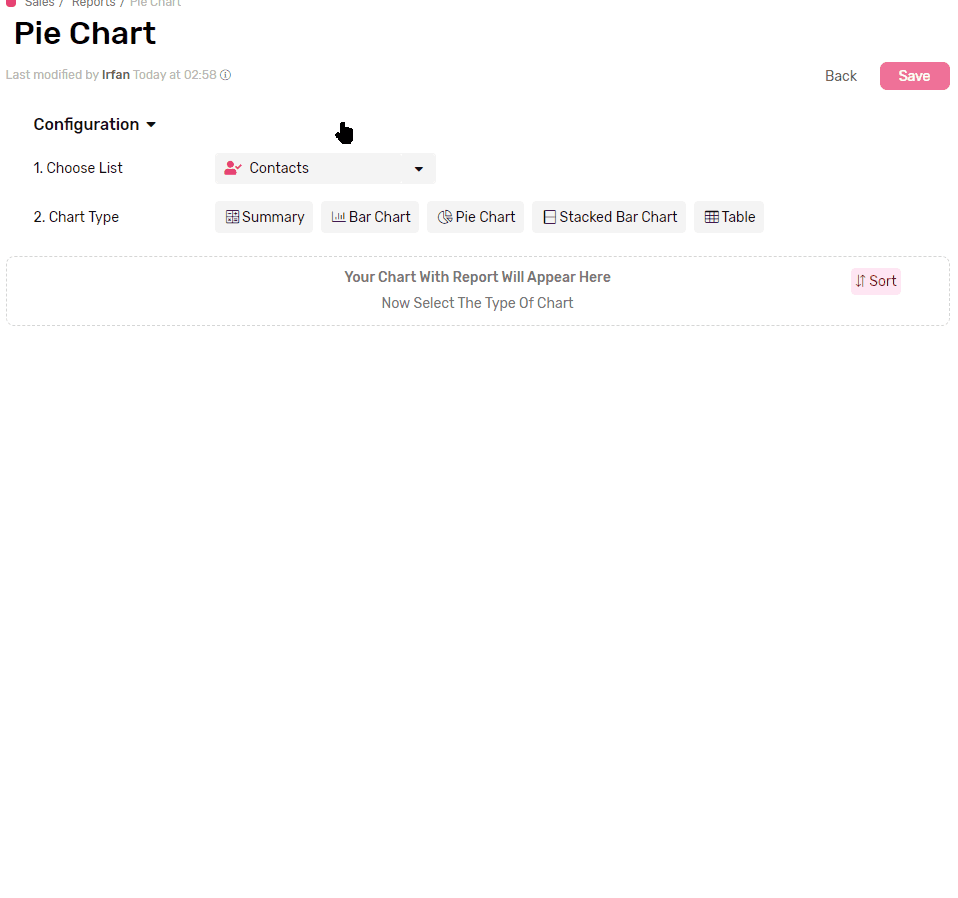## Functions

Applying a mathematical function to all values in each group, e.g. get a total of all values, applying a filter later in the configuration will limit the function to work only on the values from records which match the filter.

• Sum will calculate the total of all values in each group.
• Average will calculate the mathematical average of all values in each group.
• Max will retrieve the Maximum value in each group
• Min will retrieve the Minimum value in each group
• Count will count the records which has a value in the selected field
• Count is the only function that accepts all types of fields.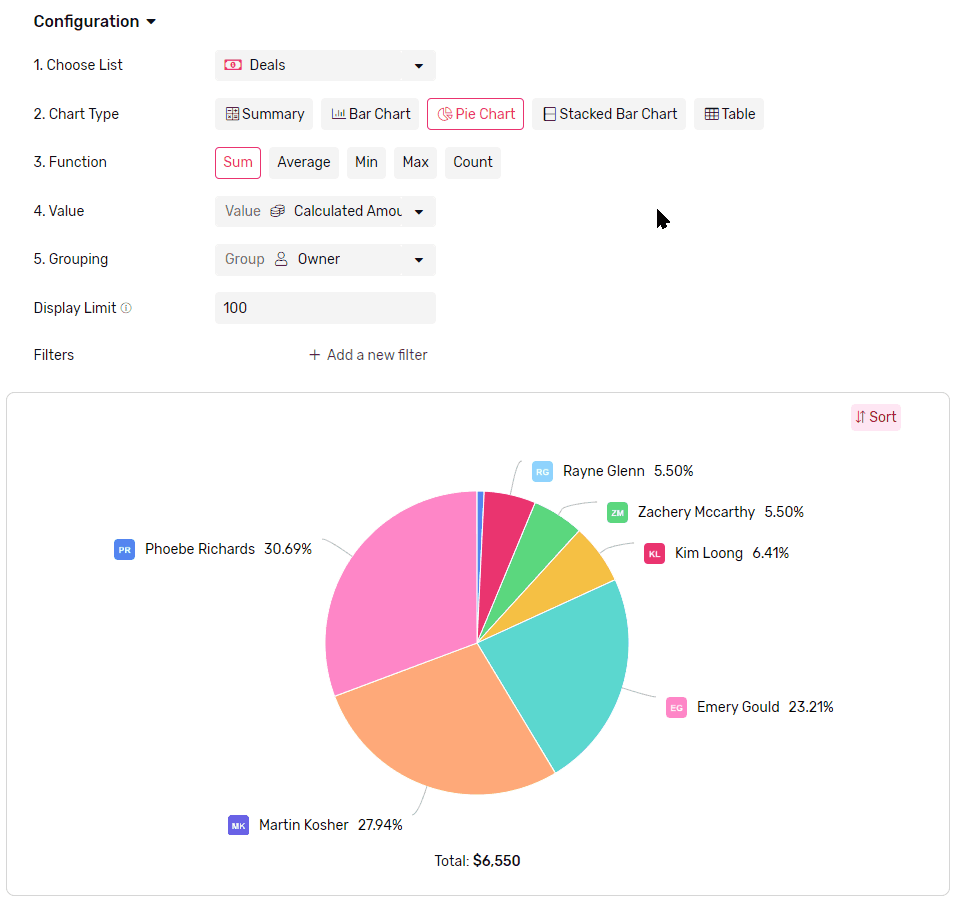## Value

• This is where you select the field that you want the function to run on.

## Grouping

• Select a field to group the data by, each group will be represented by a slice.
•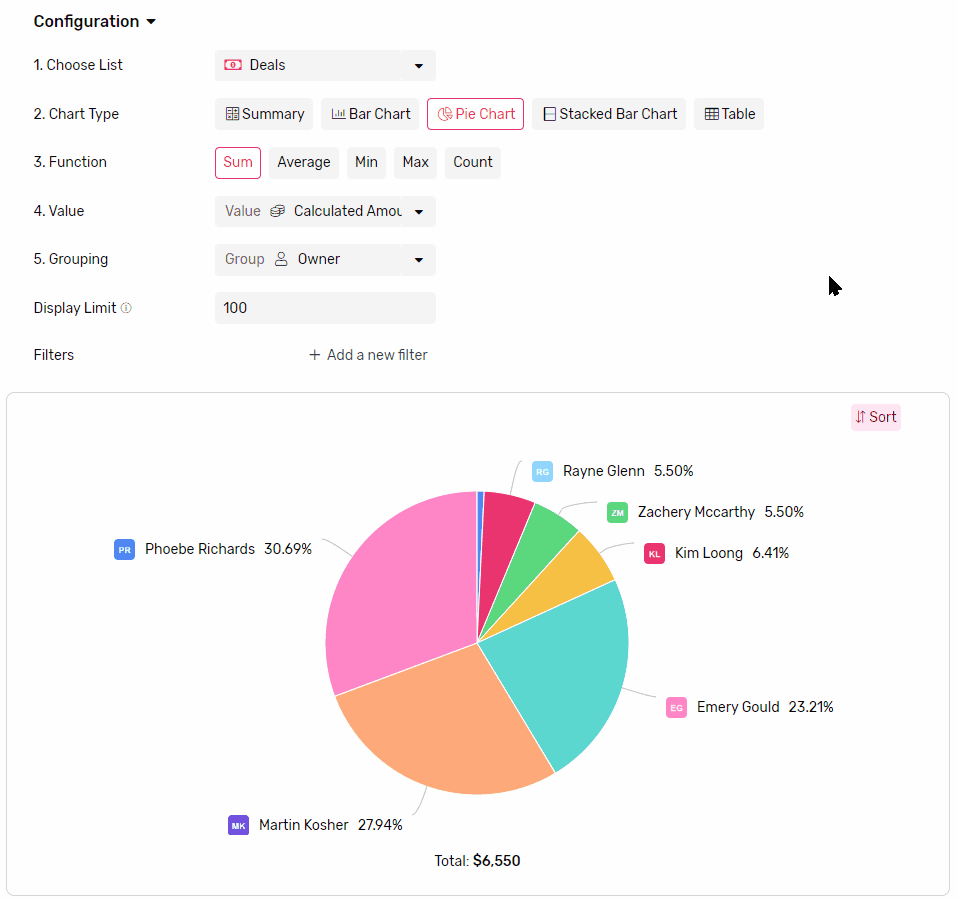• If you groupe by a date field you can also set frequency
• Frequency can Daily, Weekly, Monthly or Yearly
• Each slice will represent a time frame determined by the frequency (e.g. if you select weekly, each slice will represent a week.)
•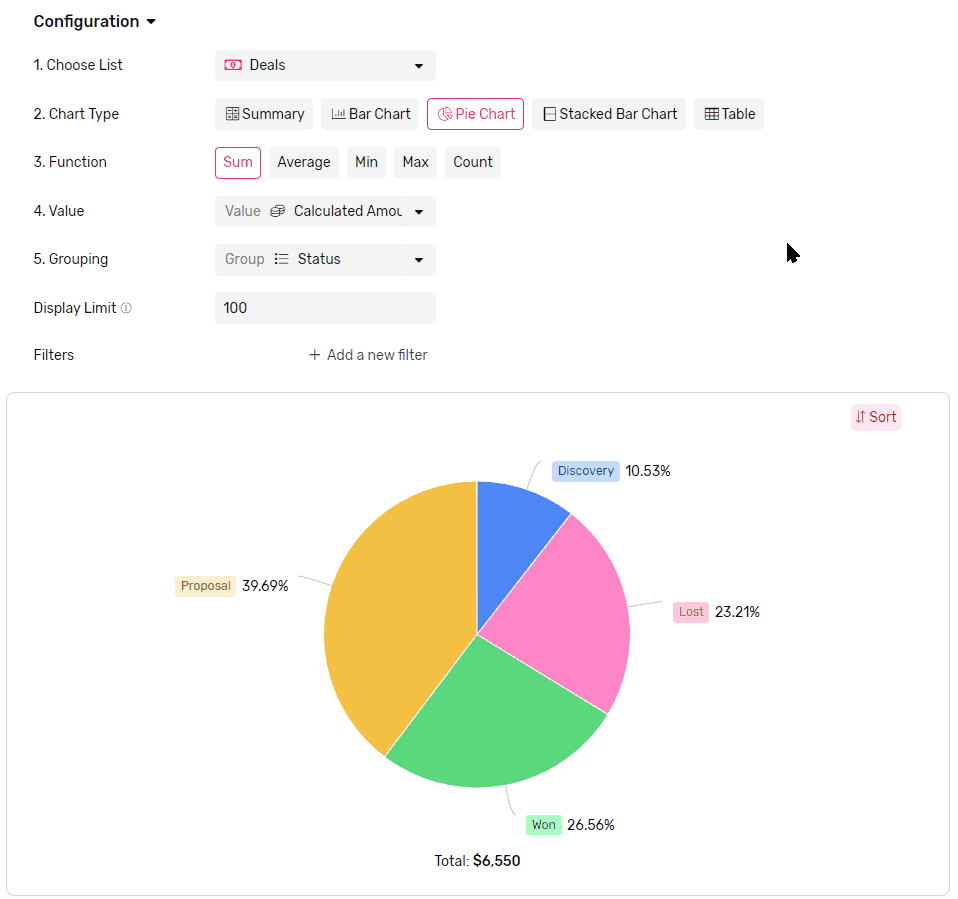## Display Limit

• Limit how many slices are displayed in the chart.
• The maximum number of slices you can see in the chart at a time is 100.
• If there are more groups than the limit the rest of the groups will be all gathered under one slice called others.
• To better control which slices are showing you can use a mix of Filters and Sorting.
•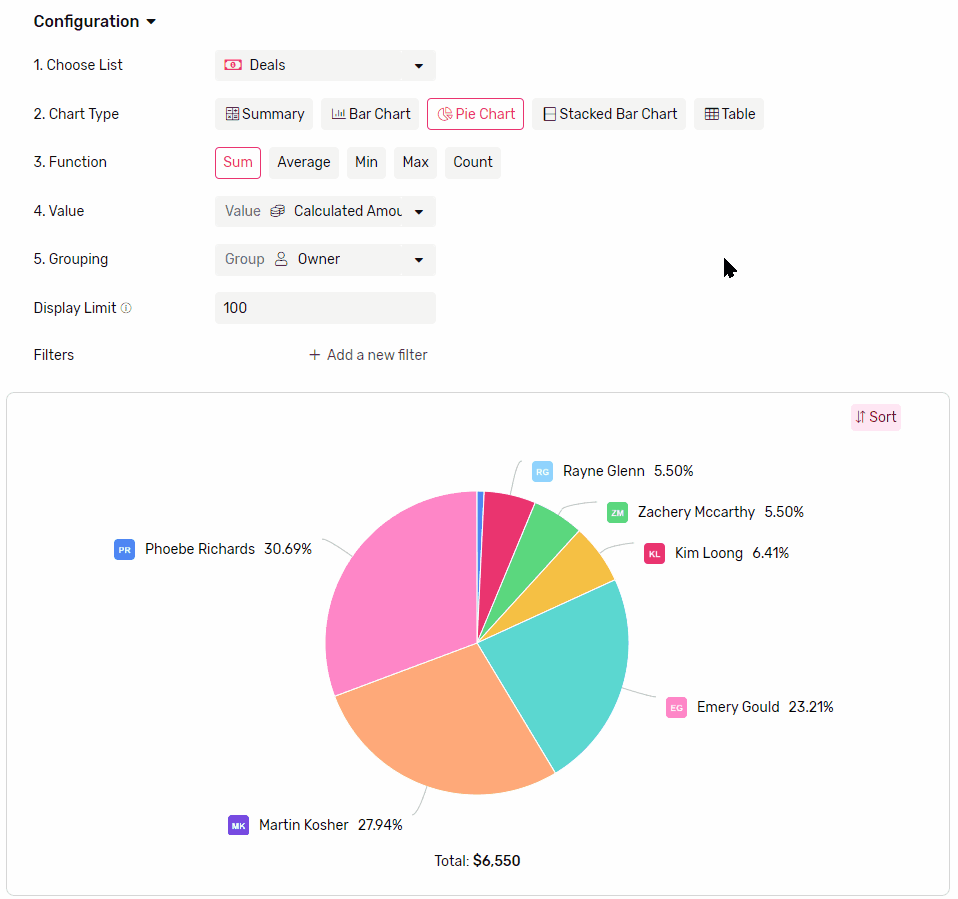## Sorting

• You can sort your slices either by
• Value, which will sort based on comparing the value represented in the slices.
• Legend, which will sort based on groups labels.
•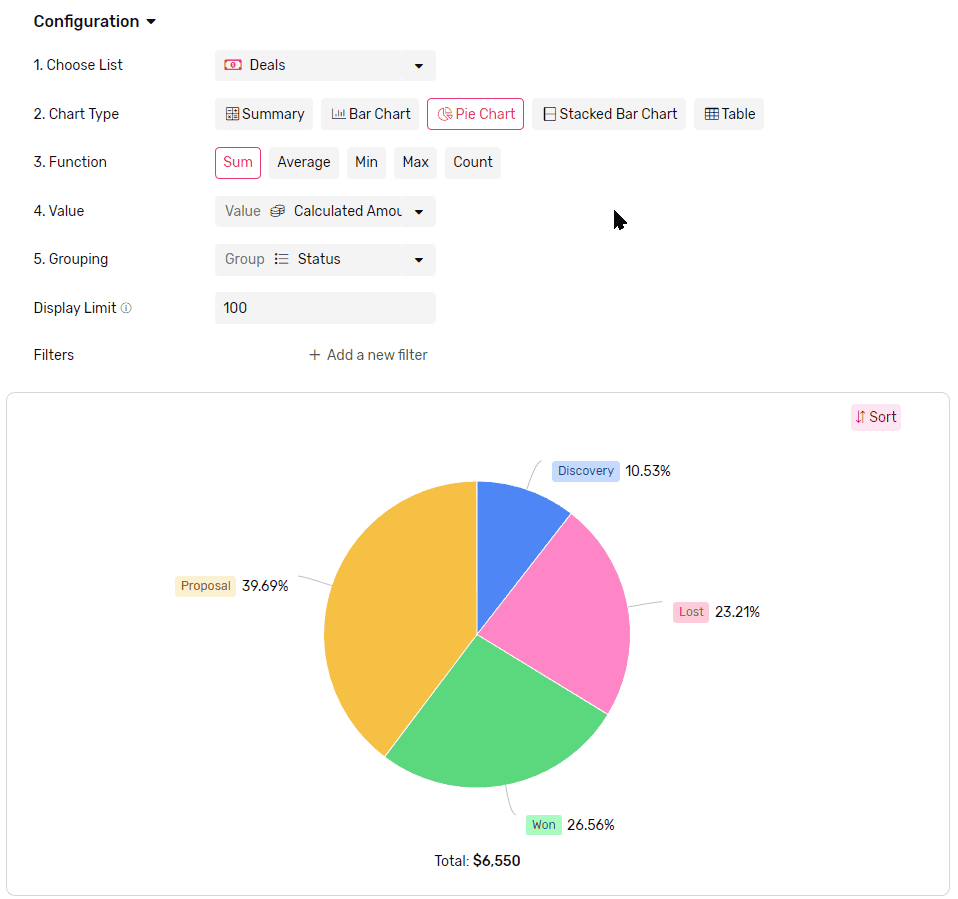### Looking for something else?

Search by entering some keywords such as; 'email automation', 'linked list'...